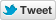# How Many Atoms Are In the Observable Universe?Long-time Slashdot reader fahrbot-bot quotes LiveScience’s exploration of the math:

To start out ‘small,’ there are around 7 octillion, or 7×10^27 (7 followed by 27 zeros), atoms in an average human body, according to The Guardian. Given this vast sum of atoms in one person alone, you might think it would be impossible to determine how many atoms are in the entire universe. And you’d be right: Because we have no idea how large the entire universe really is, we can’t find out how many atoms are within it.

However, it is possible to work out roughly how many atoms are in the observable universe — the part of the universe that we can see and study — using some cosmological assumptions and a bit of math.

[…]

Doing the math

To work out the number of atoms in the observable universe, we need to know its mass, which means we have to find out how many stars there are. There are around 10^11 to 10^12 galaxies in the observable universe, and each galaxy contains between 10^11 and 10^12 stars, according to the European Space Agency. This gives us somewhere between 10^22 and 10^24 stars. For the purposes of this calculation, we can say that there are 10^23 stars in the observable universe. Of course, this is just a best guess; galaxies can range in size and number of stars, but because we can’t count them individually, this will have to do for now.

On average, a star weighs around 2.2×10^32 pounds (10^32 kilograms), according to Science ABC, which means that the mass of the universe is around 2.2×10^55 pounds (10^55 kilograms). Now that we know the mass, or amount of matter, we need to see how many atoms fit into it. On average, each gram of matter has around 10^24 protons, according to Fermilab, a national laboratory for particle physics in Illinois. That means it is the same as the number of hydrogen atoms, because each hydrogen atom has only one proton (hence why we made the earlier assumption about hydrogen atoms).

This gives us 10^82 atoms in the observable universe. To put that into context, that is 100,000,000,000,000,000,000,000,000,000,000,000,000,000,000,000,000,000,000,000,000,000,000,000,000,000,000,000 atoms.

This number is only a rough guess, based on a number of approximations and assumptions. But given our current understanding of the observable universe, it is unlikely to be too far off the mark.

Read more of this story at Slashdot.